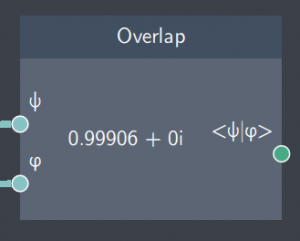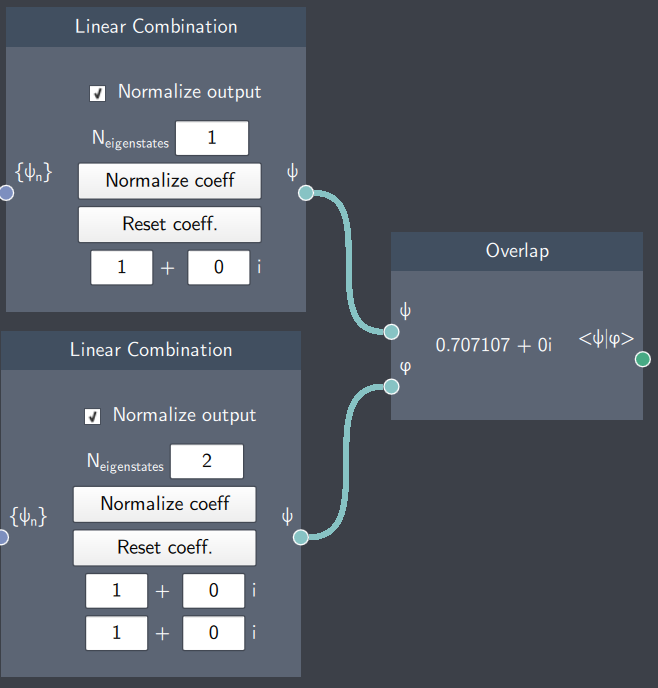# Overlap

## Description

This node calculates the overlap $\langle \psi| \varphi\rangle$ between any two states $\psi$ and $\varphi$.## Input

The node has the following inputs:

• $\psi$: State 1
• : State 2

## Content

This node displays the overlap between any two states provided as inputs.

## Output

• Overlap $\langle \psi| \varphi\rangle$: The overlap of the states provided as inputs ($\psi$ and $\varphi$).

## Example

In the example below, the overlap between a stationary state and a non-stationary state (given by a linear combination of the first two eigenstates) is calculated by the Overlap node.